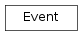# mvpa2.misc.data_generators.Event¶class `mvpa2.misc.data_generators.``Event`(**kwargs)

Simple class to define properties of an event.

The class is basically a dictionary. Any properties can be passed as keyword arguments to the constructor, e.g.:

```>>> ev = Event(onset=12, duration=2.45)
```

Conventions for keys:

`onset`
The onset of the event in some unit.
`duration`
The duration of the event in the same unit as `onset`.
`label`
E.g. the condition this event is part of.
`chunk`
Group this event is part of (if any), e.g. experimental run.
`features`
Any amount of additional features of the event. This might include things like physiological measures, stimulus intensity. Must be a mutable sequence (e.g. list), if present.

Methods

 `as_descrete_time`(dt[, storeoffset, offsetattr]) Convert `onset` and `duration` information into descrete timepoints. `clear`(() -> None.  Remove all items from D.) `copy`(() -> a shallow copy of D) `fromkeys`(...) v defaults to None. `get`((k[,d]) -> D[k] if k in D, ...) `has_key`((k) -> True if D has a key k, else False) `items`(() -> list of D’s (key, value) pairs, ...) `iteritems`(() -> an iterator over the (key, ...) `iterkeys`(() -> an iterator over the keys of D) `itervalues`(...) `keys`(() -> list of D’s keys) `pop`((k[,d]) -> v, ...) If key is not found, d is returned if given, otherwise KeyError is raised `popitem`(() -> (k, v), ...) 2-tuple; but raise KeyError if D is empty. `setdefault`((k[,d]) -> D.get(k,d), ...) `update`(([E, ...) If E present and has a .keys() method, does: for k in E: D[k] = E[k] `values`(() -> list of D’s values) `viewitems`(...) `viewkeys`(...) `viewvalues`(...)
Parameters: **kwargs : dict All keys to describe the Event to initialize its dict.

Methods

 `as_descrete_time`(dt[, storeoffset, offsetattr]) Convert `onset` and `duration` information into descrete timepoints. `clear`(() -> None.  Remove all items from D.) `copy`(() -> a shallow copy of D) `fromkeys`(...) v defaults to None. `get`((k[,d]) -> D[k] if k in D, ...) `has_key`((k) -> True if D has a key k, else False) `items`(() -> list of D’s (key, value) pairs, ...) `iteritems`(() -> an iterator over the (key, ...) `iterkeys`(() -> an iterator over the keys of D) `itervalues`(...) `keys`(() -> list of D’s keys) `pop`((k[,d]) -> v, ...) If key is not found, d is returned if given, otherwise KeyError is raised `popitem`(() -> (k, v), ...) 2-tuple; but raise KeyError if D is empty. `setdefault`((k[,d]) -> D.get(k,d), ...) `update`(([E, ...) If E present and has a .keys() method, does: for k in E: D[k] = E[k] `values`(() -> list of D’s values) `viewitems`(...) `viewkeys`(...) `viewvalues`(...)
`as_descrete_time`(dt, storeoffset=False, offsetattr='offset')

Convert `onset` and `duration` information into descrete timepoints.

Parameters: dt : float Temporal distance between two timepoints in the same unit as `onset` and `duration`. storeoffset : bool If True, the temporal offset between original `onset` and descretized onset is stored as an additional item. offsetattr : str The name of the attribute that is used to store the computed offset in case the `storeoffset` is enabled. A copy of the original `Event` with `onset` and optionally `duration` replaced by their corresponding descrete timepoint. The new onset will correspond to the timepoint just before or exactly at the original onset. The new duration will be the number of timepoints covering the event from the computed onset timepoint till the timepoint exactly at the end, or just after the event. Note again, that the new values are expressed as #timepoint and not in their original unit!